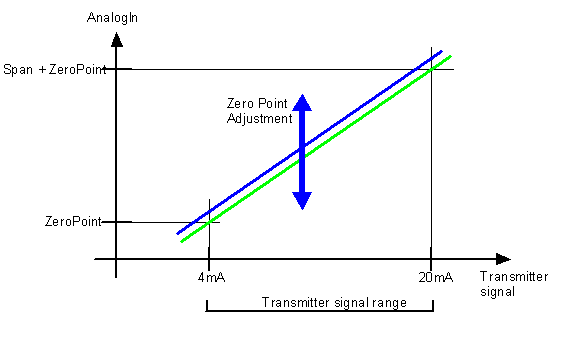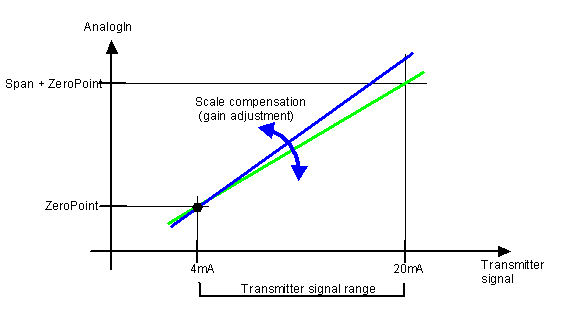## Transmitter error compensation

Adjusting the Zero Point and Span constants compensates for errors in a transmitter, due to offset drift or gain drift.

The best compensation is achieved when the transmitter signal is close to a minimum or maximum signal.
The ZeroPoint should be adjusted when the transmitter signal is at a minimum.
The Span should be adjusted with a maximum signal

Drift (offset) is compensated by changing the ZeroPoint constant. This change does not influence the gain (Span). A ZeroPoint adjustment simply moves the measuring rangeCalculation of new ZeroPoint:

When the signal is low and the exact value of the object being measured is known, the new ZeroPoint can be calculated by this formula:

## New_ZeroPoint = ZeroPoint + (Exact Value - AnalogIn)

This re-calibration can be performed manually or by the controller in a situation when the exact process value is known. This could, for example, be ZeroPoint adjustment of a tank contents transmitter when the tank is empty and cleaned. The exact value in this situation would be 0 litres.

Gain error is compensated by changing the Span constant. This change does not influence the Zero Point. A Span adjustment simply changes the gain factor (slope of the graph).Calculation of new Span:
When the signal is high and the exact value of the object being measured is known, the new Span can be calculated by this formula:

## New_Span = Span * (Exact Value / AnalogIn)

This re-calibration can be performed manually or by the controller, in a situation when the exact process value is known. This could, for example, be Span adjustment of a tank contents transmitter when a high level switch is reached, or when a known amount has been transferred into the tank. The exact value in this situation would be X litres.

## Related Topics

Transmitter installation